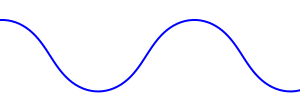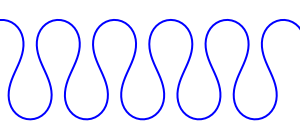## Monday, July 7, 2008

### A River Runs Through It

This week I did a bit more work on random city map generation.

I'd like this kind of city to be in a floodplain with a meandering river running through it, perhaps joining with another river. Accordingly I decided to start with the river and build up from there.

Random river construction is not something that is well-documented on the web. Luckily I know Dr. J. Wesley Lauer at Seattle University, who specializes in the interaction between rivers and their floodplains. He's given me a couple of leads to follow.

The simplest model of a meandering river is a sine-generated curve, also known as a meander curve. The river direction oscillates via a sine wave as a function of arc length. Scaling the direction angle results in a river that meanders more or less. Here are some examples (maximum angles of 1.0, 1.5, and 2.0 respectively):Here's an example with a varying direction angle scale:It's really easy to generate these. Here's some Python code I used to generate the SVG diagrams above:

``from math import sin, cosviewSizeX = 300viewSizeY = 300x = 0y = 20angle = 0angle_inc = 0.1dir_scale = 1.0step_size = 4.0points = [(x, y)]while x < viewSizeX:        dir_angle = dir_scale * sin(angle)        dir_x = step_size * cos(dir_angle)        dir_y = step_size * sin(dir_angle)        angle += angle_inc        x += dir_x        y += dir_y        points.append((x, y))print '<?xml version="1.0" encoding="UTF-8" ?>'print '<svg xmlns="http://www.w3.org/2000/svg" xmlns:xlink="http://www.w3.org/1999/xlink" width="%d" height="%d">' % (viewSizeX, viewSizeY)print '  <g fill="none" stroke="blue" stroke-width="2">'print '    <polyline points="%s"/>' % (' '.join(map(lambda p: '%g,%g' % (p, p), points)))print '  </g>'print '</svg>'``

The next step is to replace my rather-lame first attempt at river shaping with something based on sine-generated curves. Here's what I'd be replacing:The rivers in this version are based on some quadratic curves. The buildings are just splattered down in such a way as to not overlap; that'll be replaced with an algorithm that generates streets and city blocks and then subdivides the blocks into buildings. There's a bunch of little green lines which represent a gradient estimate, which I may use to guide some of the roads.